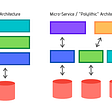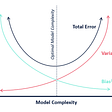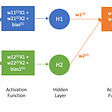# [Behind the ML] Principal Component Analysis II.

`import numpy as npimport pandas as pdimport matplotlib.pyplot as pltimport tensorflow as tf`
`CSV_COLUMN_NAMES = [‘SepalLength’, ‘SepalWidth’, ‘PetalLength’ , ‘PetalWidth’, ‘Species’]SPECIES = [‘Setosa’, ‘Versicolor’, ‘Virginica’]`
`train_path = tf.keras.utils.get_file("iris_training.csv", "https://storage.googleapis.com/download.tensorflow.org/data/iris_training.csv")test_path = tf.keras.utils.get_file("iris_test.csv", "https://storage.googleapis.com/download.tensorflow.org/data/iris_test.csv")train = pd.read_csv(train_path, names=CSV_COLUMN_NAMES, header=0)test = pd.read_csv(test_path, names=CSV_COLUMN_NAMES, header=0)train.head()train_y = train.pop('Species')test_y = test.pop('Species')# The label column has now been removed from the features.train.head()`

## 0. Exploratory Data Analysis with simple data visualizations

`import seaborn as snsimport matplotlib.pyplot as plt%matplotlib inlinesns.pairplot(train,hue=’Species’,size=2.6)`
`train_y = train.pop(‘Species’)test_y = test.pop(‘Species’)# The label column has now been removed from the features.train.head()`

## 1. Normalize

`from sklearn.preprocessing import StandardScaler X_std = StandardScaler().fit_transform(x)`
`x_scaled = (x-x.mean(axis = 0))/x.std(axis = 0)`

## 2. Eigendecomposition

• 1) Mean value of the scaled data
• 2) The dot product between the transpose of (x_scaled — mean)and (x_scaled — mean)
• 3) Dividing the value into (n-1), and ’n’ is the number of units(rows) in x_scaled, which can be reached with python code “x_scaled.shape”.
`mean_vec = np.mean(x_scaled, axis = 0) # 1dotP = (x_scaled — mean_vec).T.dot(x_scaled-mean_vec) # 2n = x_scaled.shape # 3cov_mat = dotP / (n-1)`
`eig_vals, eig_vecs = np.linalg.eig(cov_mat)`
`u,s,v = np.linalg.svd(x_scaled.T)u`

## 3. Sorting out principal components

• 1) (eigenvalue, eigenvector) pair, let’s name this as ‘eigen_pair’.
• 2) Sorting out the ‘eigen_pair’ depending on the eigenvalue which each can be reached with the code ‘eigen_pair’.
• 3) A total sum of eigenvalues
• 4) Percentage calculation to show how much variance has been explained with the eigenvector
`eig_pairs = [(np.abs(eig_vals[i]), eig_vecs[:,i]) for i in range(len(eig_vals))] # 1eig_pairs.sort(key=lambda x: x, reverse=True) # 2total_sum = sum(eig_vals) # 3variance_explained = [(i/total_sum)*100 for i in sorted(eig_vals, reverse = True)] # 4`

## 4. Projection Matrix

• 1) The first eigenvector with the highest eigenvalue
• 2) The second-highest eigenvalue’s eigenvector
• 3) Concatenating the two eigenvectors column-wise, more powerful one comes first.
`the_highest = eig_pairs # 1second_highest = eig_pairs # 2matrix_w = np.hstack((the_highest.reshape(4,1),second_highest.reshape(4,1)))# 3`

## 5. Migration from the existing data space into the newly constructed feature space using the projection matrix

`y = x_scaled.dot(matrix_w)`

--

--

--

## More from A Ydobon

Ydobon is nobody.

Love podcasts or audiobooks? Learn on the go with our new app.

## Polylithic Data Architecture## What Exactly is Data Stewardship and Why Do You Need It?## Algorithmic Trading using Sentiment Analysis on r/WallStreetBets## Turn it around — could businesses sell data to consumers?## Road Map to Data Science## 10 Must-Know Plots in Data Science## A Ydobon

Ydobon is nobody.

## What exactly is a back propagation technique?## Part 3 : Percentages, Percentiles and Quartiles## A Silent Killer in India: Air Pollution## To Cat or to not cat: A beginner’s guide to implementing a logistic regression algorithm for image…